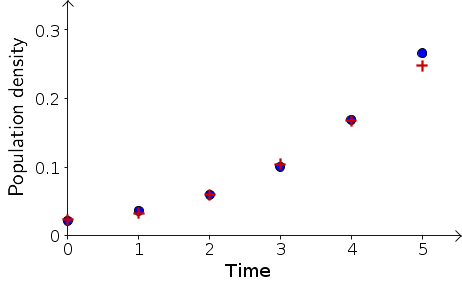# Math Insight

### Image: Comparing evolution of bacteria population density with a parabola modelExperimental data from measurements of bacteria population density are compared with a model where the population size is a quadratic function of time. The quadratic model $B_t= 0.0236 + .000186t + 0.00893 t^2$ was determined by fitting a parabola to the data points. The data was taken from the following table with time rescaled so that each snapshot was one unit of time.

Measurements of bacterial density at pH 6.25.
The units of “Population Density” are those of absorbance as measured by a spectrophotometer.
Time (min)Time indexPopulation Density
000.022
1610.036
3220.060
4830.101
6440.169
8050.266

Image file: bacteria_density_versus_time_parabola.png

Source image file: bacteria_density_versus_time_parabola.ggb
Source image type: Geogebra